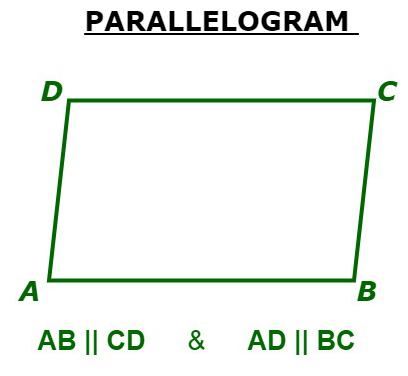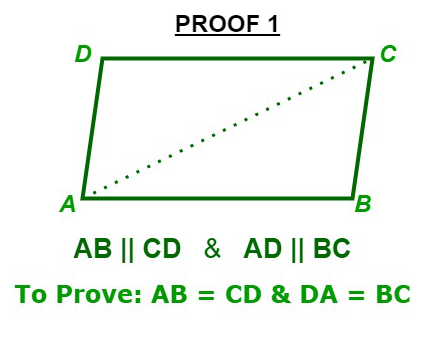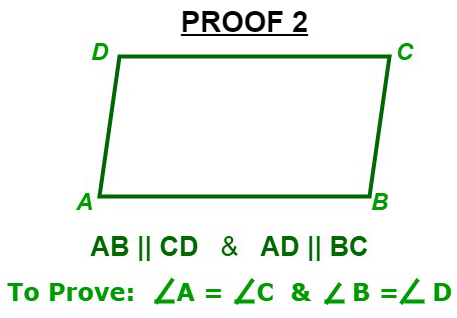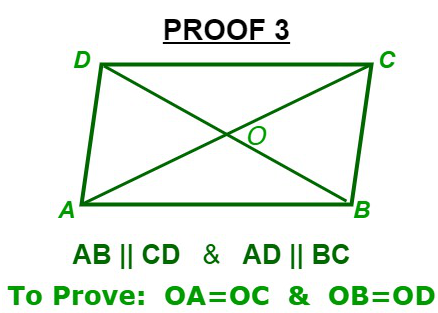# Properties of Parallelograms

• Last Updated : 02 Sep, 2021

A quadrilateral having both the pairs of opposite sides equal is a parallelogram. A parallelogram is a two-dimensional geometrical shape, whose sides are parallel to each other. Below are some simple facts about parallelogram:

1. Number of sides in Parallelogram = 4
2. Number of vertices in Parallelogram = 4
3. Area = Base x Height
4. Perimeter = 2 (Sum of adjacent sides length)
5. Type of polygon = Quadrilateral

Below is the representation of a parallelogram:

Hey! Looking for some great resources suitable for young ones? You've come to the right place. Check out our self-paced courses designed for students of grades I-XII

Start with topics like Python, HTML, ML, and learn to make some games and apps all with the help of our expertly designed content! So students worry no more, because GeeksforGeeks School is now here!## Proofs: Parallelograms

### Proof 1: Opposite sides of a parallelogram is equal.Given: ABCD is a parallelogram

To Prove: AB = CD & DA = BC

Firstly, Join AC

As given ABCD is a parallelogram. Therefore,

AB || DC  &  AD || BC

Now,  AD || BC and AC is intersecting A and C respectively.DAC =BCA                   …(i)                  [Alternate Interior Angles]

Now, AB || DC and AC is intersecting A and C respectively.BAC =DCA                 …(ii)                      [Alternate Interior Angles]

Now, InADC &CBADAC =BCA                    [ From (i) ]

AC = AC                                   [ Common Side ]DCA =BAC                 [ From (ii) ]

So, by ASA(Angle-Side-Angle) criterion of congruenceADCCBA

AB = CD & DA = BC [ Corresponding part of congruent triangles are equal ]

Hence Proved !

### Proof 2: Opposite angles of a parallelogram are equal.Given: ABCD is a parallelogram

To Prove:A =C  andB =D

As given ABCD is a parallelogram. Therefore,

AB || DC  &  AD || BC

Now, AB || DC and AD is Intersecting them at A and D respectively.A +D = 180…(i)             [ Sum of consecutive interior angles is 180]

Now, AD || BC and DC is Intersecting them at D and C respectively.D +C = 180…(ii)            [ Sum of consecutive interior angles is 180\degree]

From (i) and (ii) , we getA +D =D  +C

So,A =C

Similarly,B =DA =C andB =D

Hence Proved !

### Proof 3: Diagonals of a parallelogram bisect each other.Given: ABCD is a parallelogram

To Prove: OA = OC & OB = OD

As given ABCD is a parallelogram. Therefore,

AB || DC  &  AD || BC

Now, AB || DC and AC is intersecting A and C respectively.BAC =DCA                               [ Alternate Interior Angles are equal ]

So,BAO =DCO

Now,  AB || DC and BD is intersecting B and D respectively.ABD =CDB                               [ Alternate Interior Angles are equal ]

So,ABO =CDO

Now, inAOB &COD we have,BAO =DCO                               [ Opposite sides of a parallelogram are equal ]

AB = CDABO =CDO

So, by ASA(Angle-Side-Angle) congruence criterionAOBCOD

OA = OC and OB = OD

Hence Proved !

My Personal Notes arrow_drop_up